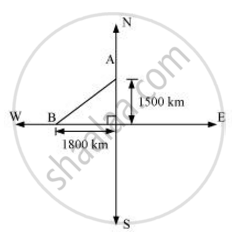# An aeroplane leaves an airport and flies due north at a speed of 1,000 km per hour. At the same time, another aeroplane leaves the same airport and flies due west at a speed of 1,200 km per hour. - Mathematics

An aeroplane leaves an airport and flies due north at a speed of 1,000 km per hour. At the same time, another aeroplane leaves the same airport and flies due west at a speed of 1,200 km per hour. How far apart will be the two planes after 1 1/2 hours?

#### SolutionDistance travelled by the plane flying towards north in 1 1/2

Similarly, distance travelled by the plane flying towards west in 1 1/2

Let these distances be represented by OA and OB respectively.

Applying Pythagoras theorem,

Distance between these planes after 1 1/2

= (sqrt((1500)^2 + (1800)^2))

= (sqrt5490000)

Therefore, the distance between these planes will be 300sqrt(61)

Concept: Right-angled Triangles and Pythagoras Property
Is there an error in this question or solution?

#### APPEARS IN

NCERT Class 10 Maths
Chapter 6 Triangles
Exercise 6.5 | Q 11 | Page 151